The Definition of a Triangle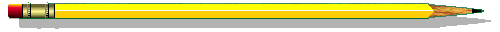Examples of Triangles

A triangle is a special kind of shape.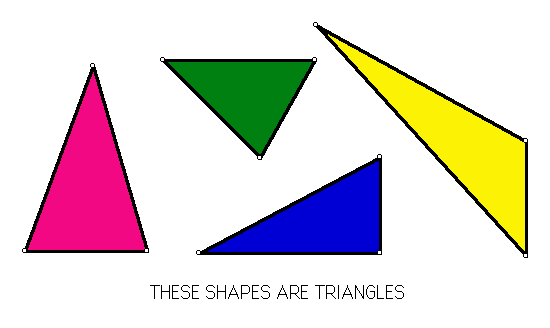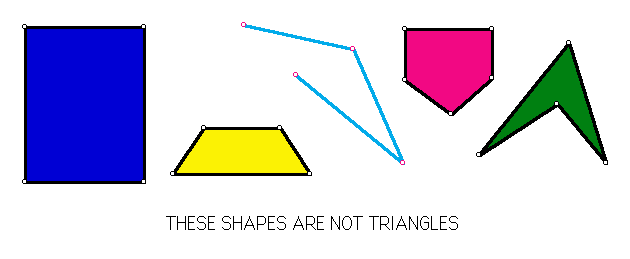What do you think makes a shape a triangle?

Be sure to think about this question before going on with the lessonProperties of triangles

Before we decide exactly what makes a shape a triangle, we need to understand what a closed figure is.

A figure is closed if all its sides are connected.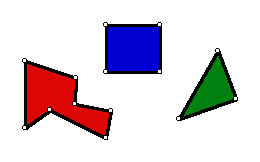These figures are closed. These figures are not closed.

A triangle is a closed figure with three sides.

Use this definiton to figure out which of the following shapes are triangles and which are not.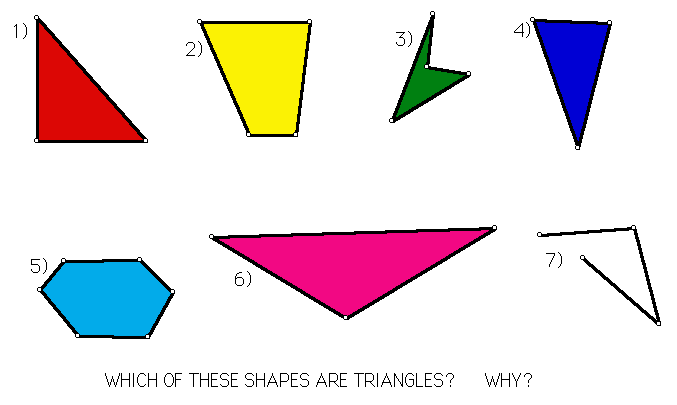Click here to check your answers.

Can you think of any triangles that you see in your everyday life?

(Hint: look at bridges or radio towers.)Classification of Triangles

There are many different kinds of triangles.

The following table outlines some basic types of triangles.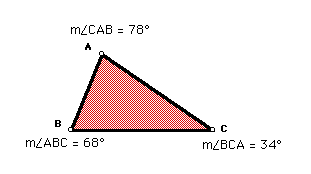Acute trianlges are triangles in which the measures of all three angles are less than 90 degrees.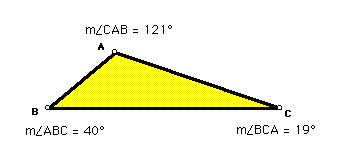Obtuse triangles are triangles in which the measure of one angle is greater than 90 degrees.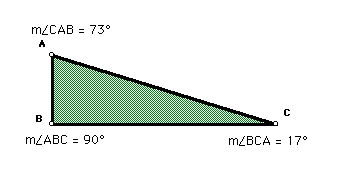Right triangles are triangles in which the measure of one angle equals 90 degrees.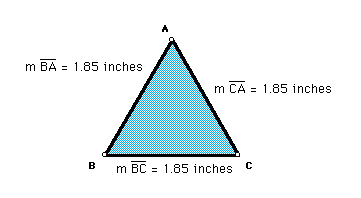Equilateral triangles are triangles in which all three sides are the same length.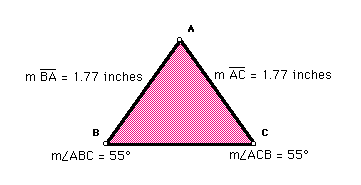Isosceles triangles are triangles in which two of the sides are the same length.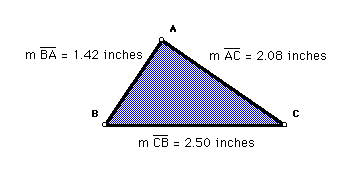Scalene triangles are triangles in which none of the sides are the same length.Triangles: Everything you ever wanted to know about them

 The Definition of a Triangle The Area of a Triangle The Sum of the Angles of a Triangle Right Triangles Triangulation The Stability of a Triangle The Rigidity of Triangles Building Bridges Links To Related Websites